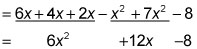##### Basic Math and Pre-Algebra Workbook For Dummies, with Online Practice, 3rd EditionWhen an expression has two sets of parentheses next to each other, you need to multiply every term inside the first set of parentheses by every term in the second set. This process is called FOILing.

The word FOIL is a memory device for the words First, Outside, Inside, Last, which helps keep track of the multiplication when both sets of parentheses have two terms each.

## Practice questions

1. Simplify the expression (x + 7)(x – 2).
2. Simplify the expression 6x – (x – 2)(x – 4) + 7x2.

1. The answer is x2 + 5x – 14.

Begin by FOILing the parentheses. Start by multiplying the two first terms: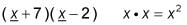Multiply the two outside terms: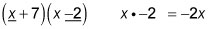Multiply the two inside terms: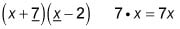Finally, multiply the two last terms: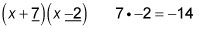Add these four products together and simplify by combining like terms: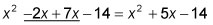2. The answer is 6x2 + 12x – 8.

Begin by FOILing the parentheses: Multiply the first, outside, inside, and last terms: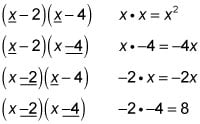Add these four products together and put the result inside one set of parentheses, replacing the two sets of parentheses that were originally there:

6x – (x – 2)(x – 4) + 7x2 = 6x – (x2 – 4x – 2x + 8) + 7x2

The remaining set of parentheses is preceded by a minus sign, so change the sign of every term in there to its opposite and drop the parentheses:

= 6xx2 + 4x + 2x – 8 + 7x2

Now simplify the expression by combining like terms and reordering your solution: